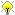# Elliptic Curves: Specific Task List

Let a be a root of x^2-x-1.

## Specific Concrete Little Questions

• Find the isogeny class of the curve [1,a+1,a,a,0].
• Data up to norm conductor 124.

## Major Projects

I keep thinking of good ideas for projects for the summer REU involving elliptic curves. Thus I'll start listing them here. -- William

• Create a SQLite version of Cremona's tables of elliptic curves. Make indexes and have it be very easy to query. Have it replace the current Cremona database in Sage.

• Work out the details of the analogue of the *statement* of the Gross-Zagier formula for elliptic curves over Q(sqrt(5)). Use this to give an algorithm to compute the height of Heegner points, hence their index [E(K): Z y_K] in the full group of rational points.

• Give the analogue of Kolyvagin's bound -- that sqrt(#Sha) divides [E(K): Z y_K] over Q(sqrt(5)). Use this to prove that some Shafarevich-Tate groups of elliptic curves over Q(sqrt(5)) are trivial. This will involve Galois cohomology and following -- perhaps word for word-- the arguments in either B. Gross or W. McCallum's articles on Kolyvagin's work.

• Create as complete as possible of tables like Cremona's electronic tables, but for elliptic curves over Q(sqrt(5)).
• Create a *print* version of tables of all curves up to norm conductor 1000 over Q(sqrt(5)), which looks just like Cremona's tables.
• Give an algorithm to find minimal twists over Q(sqrt(5)) that is exactly analogous to the one described in Section 2.1 of Stein-Watkins.

• Give an analogue of Mazur's theorem over Q(sqrt(5)). This is the theorem that classifies the prime degrees of rational isogenies. (Update: A different but related problem has been solved by Filip Najman -- personal email to wstein on June 1, 2011.) http://web.math.hr/~fnajman/skfn.pdf;

reu/2011/problems (last edited 2011-09-08 21:59:31 by was)